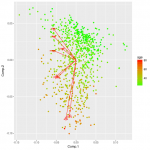Want to share your content on R-bloggers? click here if you have a blog, or here if you don't.Shiny Application Layouts-Vertical Layout

In the fifth part of our series we will apply the `kmeans()` function to the `iris` dataset to create a shiny application. The difference is that now we will display its result vertically.

This part can be useful for you in two ways.

First of all, you can see different ways to enhance the appearance and the utility of your shiny app.

Secondly you can make a revision on what you learnt in “Building Shiny App” series as we will build basic shiny staff in order to present it in the proper way.

Read the examples below to understand the logic of what we are going to do and then test yous skills with the exercise set we prepared for you. Lets begin!

Answers to the exercises are available here.

Vertical Layout

Every element passed to `verticalLayout()` will occupy a full row. The difference with `fluidRow()` is that `verticalLayout()` does not expect to be another column inside. Look at the example below:
#ui.R
`fluidPage(`

``` verticalLayout( titlePanel("example"), plotOutput("plot"), wellPanel( sliderInput("p", "Points", 10, 200, value = 50, step = 10) ) ) ```

`)`

Exercise 1

Create the initial UI. HINT: Use `fluidpage()`.

Exercise 2

Apply the vertical layout to your UI. HINT: Use `verticalLayout()`.

Exercise 3

Give the title “Vertical Iris” to your app. HINT: Use `titlePanel()`.

Exercise 4

Create the ui side of your plot. HINT: Use `plotOutput`.

You can use the `wellPanel()` function which will help you keep your elements separated. Now you should create a `selectInput()` with the X variables of the `iris` dataset.
#ui.R
`wellPanel(selectInput('x', 'X Variable', names(iris)))`

Learn more about Shiny in the online course R Shiny Interactive Web Apps – Next Level Data Visualization. In this course you will learn how to create advanced Shiny web apps; embed video, pdfs and images; add focus and zooming tools; and many other functionalities (30 lectures, 3hrs.).

Exercise 5

Put a `selectInput()` to choose the X variable under your plot. HINT: Use `selectInput()` and `wellPanel()`.

In the next two rows we will put with the same way a `selectInput()` and a `numericInput()` for the Y Variable and the number of clusters respectively, like the example below.
#ui.R
`selectInput('y', 'Y Variable', names(iris),`

``` selected=names(iris)[]), numericInput('clusters', 'ClusterS', 3, ```

` min = 1, max = 9)`

Exercise 6

Create a `selectInput()` for the Y Variable and a `numericInput()` to decide the number of clusters and separate them. The features of both are up to you.

Now we have to combine the selected variables for x and y into a new data frame like the example below:
#ui.R
```comb <- reactive({ data[, c(input\$x, input\$y)] })```

Exercise 7

Combine the selected variables into a new data frame. HINT: Use `reactive({})`.

In order to apply the `kmeans()` function and decide the number of clusters, to the new data frame we have to use reactivity again. Here is an example:
#ui.R
```clusters <- reactive({ kmeans(comb(), input\$clusters) })```

Exercise 8

Apply the `kmeans()` function to `Data()` and use as input the number of clusters. HINT: Use `reactive({})`.

Exercise 9

Plot `Data()` to activate your plot on the server side. HINT: Use `renderPlot()`.

To create a completed kmeans plot you have to add cluster centers and separate each team by color. You can do this with this part of code.
`col = clusters()\$cluster,`

``` pch = 20, cex = 3) ```

` points(clusters()\$centers, pch = 4, cex = 4, lwd = 4)`

Exercise 10

Add the code above in the correct place of your server side to complete your kmeans plot.